# JEE Main 2020 Physics Paper With Solutions Sept 6 (Shift 2)

JEE Main 2020 Paper with Solutions Physics - Shift 2 (Sept 6) is a perfect resource to ace the exams, which provide the gateway to some of the renowned institutions of the country. Due to excessive competition, students need to prepare well in advance. The solutions uploaded at BYJU’S will enable the students to divide and plan their resources in an optimal way. Practising these questions will help them understand the amount of preparation required to face the examination.

### September 6 Shift 2 - Physics

Question 1: For a plane electromagnetic wave, the magnetic field at a point x and time t is B (x, t) = [1.2 × 10-7 sin (0.5 × 103 x + 1.5 × 1011 t) k] T. The instantaneous electric field E corresponding to B is:

(speed of light c = 3 × 108 ms–1)

1. 1) E (x, t) = [36 sin (1 × 103 x + 1.5 × 1011t) i] V / m
2. 2) E (x, t) = [36 sin (0.5 × 103 x + 1.5 × 1011t) k] V / m
3. 3) E (x, t) = [36 sin (1 × 103 x + 0.5 × 1011t) j] V / m
4. 4) E (x, t) = [- 36 sin (0.5 × 103 x + 1.5 × 1011t) j] V / m

Solution:

|E| = |B| C

⇒ 1.2 × 10-7 × sin |0.5 × 103x + 1.5 × 1011t) × 3 × 108

⇒ 36 sin (0.5 × 103x + 1.5 × 1011 t)

λ → Not change → Answer 1, 3 incorrect

E and B → not same direction → 2nd incorrect

Question 2: Particle A of mass m1 moving with velocity (√3i + j) ms-1 collides with another particle B of mass m2 which is at rest initially. Let V1 and V2 be the velocities of particles A and B after collision respectively. If m1 = 2m2 and after collision V1 = (i + √3j)ms-1, the angle between V1 and V2 is:

1. 1) 105°
2. 2) 15°
3. 3) –45°
4. 4) 60°

Solution:

From momentum conservation

(2m) (√3i + j) + 0 = 2m (i + √3j) + mv2

V2 = (2 (√3 - 1)) (i - j) and V1 = i + √3j

So the angle between v1 and v2 is 105o.

Question 3: When a car is at rest, its driver sees raindrops falling on it vertically. When driving the car with speed v, he sees that raindrops are coming at an angle 60° from the horizontal. On further increasing the speed of the car to (1 + β) v, this angle changes to 45°. The value β is close to:

1. 1) 0.50
2. 2) 0.73
3. 3) 0.37
4. 4) 0.41

Solution:

Vrm = Vr - Vm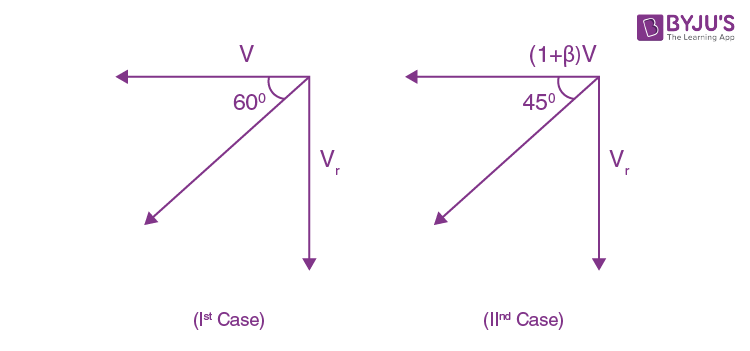tan 60 = vr / v ---- (1)

tan 45 = [vr / (1 + β) v] ----- (2)

From (1) / (2)

√3 / 1 = (1 / 4) / [(1 / (1 + β) v]

√3 = 1 + β ⇒ β = 0.732

Question 4: A charged particle going around in a circle can be considered to be a current loop. A particle of mass m carrying charge q is moving in a plane with speed v under the influence of magnetic field B. The magnetic moment of this moving particle:

1. 1) mv2B / 2B2
2. 2) - mv2B / 2πB2
3. 3) - mv2B / 2B2
4. 4) - mv2B / B2

Solution:

Magnetic dipole moment

M = iA

∴ i = qF, A = πR2

F = qB / 2πm, R = mv / qB

M = q (qB / 2πm) × π [m2v2 / q2B2]

M = mV2 / 2B

The direction of M and B is opposite.

M = mV2 / 2B . (-B)

→ [-mV2 / 2B2] B

Question 5: A double convex lens has power P and same radii of curvature R of both the surfaces. The radius of curvature of a surface of a plano-convex lens made of the same material with power 1.5 P is:

1. 1) R / 3
2. 2) 3R / 2
3. 3) R / 2
4. 4) 2R

Solution:

P = [(μl / μs) - 1)] [2 / r] ----- (1)

(3 / 2) P = [(μl / μs) - 1)] [1 / r1] ---- (2)

From (1) / (2)

[P] / [(3 / 2) P] = [2 / R] / [1 / R1]

R1 = R / 3

Question 6: A circuit to verify Ohm's law uses ammeter and voltmeter in series or parallel connected correctly to the resistor. In the circuit:

1. 1) Ammeter is always connected in series and voltmeter in parallel.
2. 2) Both, ammeter and voltmeter must be connected in series.
3. 3) Both ammeter and voltmeter must be connected in parallel.
4. 4) The ammeter is always used in parallel and voltmeter in series.

Solution:By theory in order for a voltmeter to measure a device's voltage, it must be connected in parallel to that device. This is necessary because objects in parallel experience the same potential difference.

A voltmeter measures the potential difference of the circuit and it has high internal resistance. When the voltmeter is connected in parallel with a circuit component, the amount of current passing through the voltmeter is very less. Therefore, the current through the circuit is unaltered.

In order for an ammeter to measure a device's current, it must be connected in series to that device. This is necessary because objects in series experience the same current.

Question 7: A square loop of side 2a and carrying current I is kept in the xz plane with its centre at the origin. A long wire carrying the same current I is placed parallel to the z-axis and passing through the point (0, b, 0), (b >> a). The magnitude of the torque on the loop about z-axis will be:

1. 1) 2μ0I2a2 / π b
2. 2) 2μ0I2a2b / π (a2 + b2)
3. 3) μ0I2a2 / 2π b
4. 4) μ0I2a2b / 2π (a2 + b2)

Solution: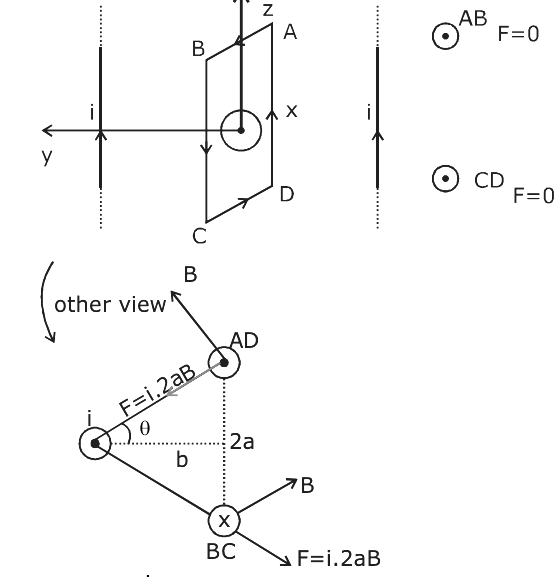B = (μ0I) / [2π √(a2 + b2)]

∴ Torque = F . cos θ . 2a

= {[i2aμ0i] / [2π √(a2 + b2)]} {[b] / [√(a2 + b2)]} . 2a

= 2μ0I2a2b / π (a2 + b2)

Question 8: In a dilute gas at pressure P and temperature T, the mean time between successive collisions of a molecule varies with T as:

1. 1) √T
2. 2) 1 / T
3. 3) T
4. 4) 1 / √T

Solution:

Vavg. ∝ √T

Tmean= λ / Vavg.

Tmean ∝ 1 / √T

Question 9: When a particle of mass m is attached to a vertical spring of spring constant k and released, its motion is described by y (t) = y0 sin2 ωt, where 'y' is measured from the lower end of unstretched spring. Then ω is:

1. 1) √g / (y0)
2. 2) (1 / 2) (√g / (y0))
3. 3) √2g / (y0)
4. 4) √g / (2y0)

Solution: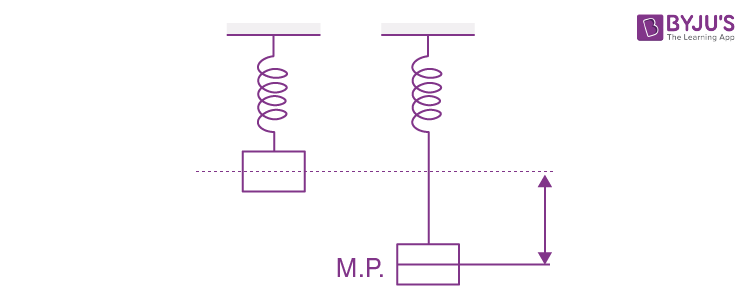y (t) = [y0 / 2] [1 - cos (2ωt)]

From comparing standard equation of SHM Amplitude A = y0 / 2

At equilibrium situation mg / k = y0 / 2

2g / y0 = k / m

2ω = √k / m

ω = (1 / 2) √k / m

ω = (1 / 2) √2g / y0

ω = √g / (2y0)

Question 10: The linear mass density of a thin rod AB of length L varies from A to B as λ (x) = λ0 [1 + (x / L)], where x is the distance from A. If M is the mass of the rod then its moment of inertia about an axis passing through A and perpendicular to the rod is:

1. 1) (2 / 5) ML2
2. 2) (5 / 12) ML2
3. 3) (7 / 18) ML2
4. 4) (3 / 7) ML2

Solution:

dm = λ0 [1 + (x / L)] dx

0M dm = ∫0L λ0 [1 + (x / L)] dx

M = 3λ0L / 2 ----- (1)dI = dm x2

∫dI = dm x2

I = ∫0L λ0 [1 + (x / L)] dx x2

I = 7λ0L3 / 12

From (1), λ0 = 2M / 3L

I = (7 / 18) ML2

Question 11: A fluid is flowing through a horizontal pipe of varying cross-section, with speed v ms–1 at a point where the pressure is P pascal. At another point where pressure is P / 2 Pascal its speed is V ms–1. If the density of the fluid is kg m–3 and the flow is streamlined, then V is equal to:

1. 1) √[(P / 2ρ) + v2]
2. 2) √[(P / ρ) + v2]
3. 3) √[(2P / ρ) + v2]
4. 4) √[(P / ρ) + v]

Solution:

From Bernoulli's equation,

P + (1 / 2) ρv2 = [P / 2] + [1 / 2] ρV12

V1 = √[(P / ρ) + v2]

Question 12: Three rods of identical cross-section and lengths are made of three different materials of thermal conductivity K1, K2 and K3, respectively. They are joined together at their ends to make a long rod (see figure). One end of the long rod is maintained at 100°C and the other at 0°C (see figure). If the joints of the rod are at 70°C and 20°C in steady-state and there is no loss of energy from the surface of the rod, the correct relationship between K1, K2 and K3 are: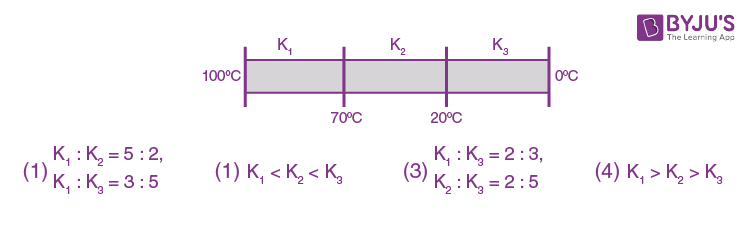Solution:

Heat current same

[K1 (100 - 70)] / R1 = [K2 (70 - 20)] / R2 = [K3 (20 - 0)] / R3

R1 = R2 = R3

30k1 = 50k2 = 20k3

K1 / k2 = 5 / 3, K2 / k3 = 2 / 5

K1 / K3 = 2 / 3

Question 13: Assuming the nitrogen molecule is moving with r.m.s. velocity at 400 K, the de-Broglie wavelength of nitrogen molecule is close to (Given: nitrogen molecule weight: 4.64 × 10–26 kg, Boltzman constant: 1.38 × 10–23 J/K, Planck constant: 6.63 × 10–34 J.s)

1. 1) 0.44 Å
2. 2) 0.34 Å
3. 3) 0.20 Å
4. 4) 0.24 Å

Solution:

λ = λ / [mvr.m.s]

∴ v = √3kT / M

λ = h / √3mkT

λ = [6.63 × 10–34] / [√3 × 4.6 × 10-26 × 1.38 × 10-23 × 400]

λ = 2.4 × 10-11

⇒ 0.24Å

Question 14: Consider the force F on a charge 'q' due to a uniformly charged spherical shell of radius R carrying charge Q distributed uniformly over it. Which one of the following statements is true for F, if 'q' is placed at distance r from the centre of the shell?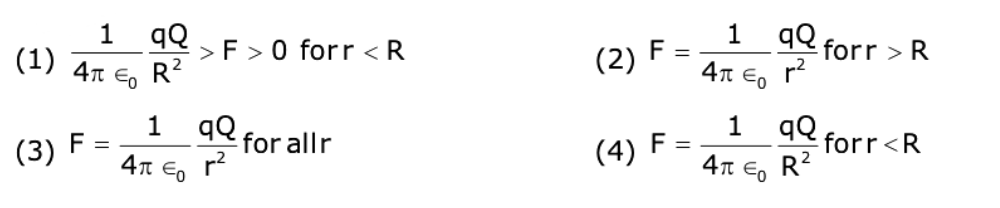Solution:

For r < R

E = 0

For r > R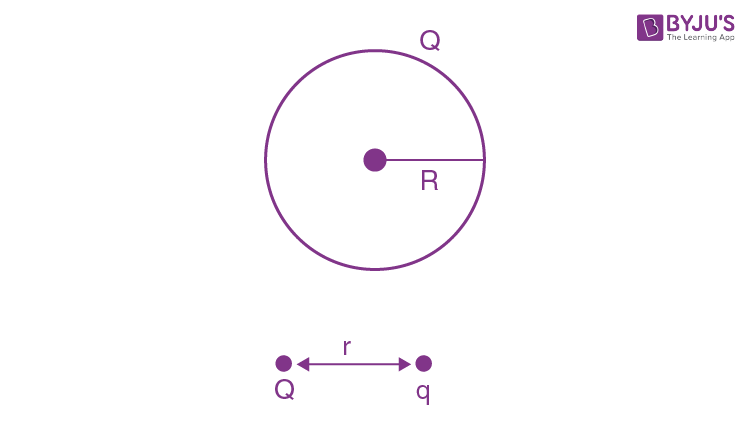F = kQq / r2

Question 15: Two identical electric point dipoles have dipole moments p1 = pi and p1 = -pi are held on the x-axis at distance 'a' from each other. When released, they move along the x-axis with the direction of their dipole moments remaining unchanged. If the mass of each dipole is 'm', their speed when they are infinitely far apart is:

1. 1)
$$\frac{p}{a}\sqrt{\frac{3}{2\pi \epsilon _{0}ma}}$$
2. 2)
$$\frac{p}{a}\sqrt{\frac{1}{\pi \epsilon _{0}ma}}$$
3. 3)
$$\frac{p}{a}\sqrt{\frac{1}{2\pi \epsilon _{0}ma}}$$
4. 4)
$$\frac{p}{a}\sqrt{\frac{2}{\pi \epsilon _{0}ma}}$$

Solution: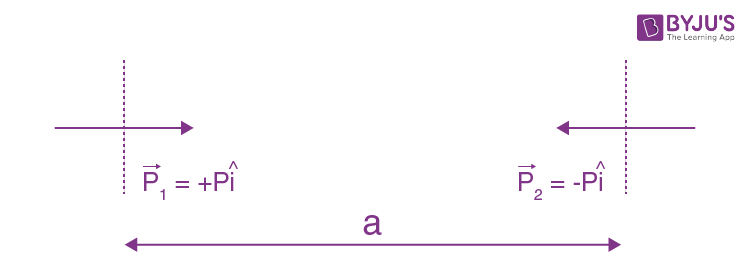The interaction energy of dipole is

= p (dv / dr) ⇒ P {[d] (Kp / r2)} / dr ⇒ -2kP / r3

Now from E.C.

[2kP / r3] = [1 / 2] mv2 + [1 / 2] mv2

V = √2kp2 / mr3

∴ r = a

$$\frac{p}{a}\sqrt{\frac{1}{2\pi \epsilon _{0}ma}}$$

Question 16: Two planets have masses M and 16 M and their radii are a and 2a, respectively. The separation between the centres of the planets is 10a. A body of mass m is fired from the surface of the larger planet towards the smaller planet along the line joining their centres. For the body to be able to reach at the surface of the smaller planet, the minimum firing speed needed is:

1. 1) 2 √GM / a
2. 2) √GM2 / ma
3. 3) (3 / 2) √5GM / a
4. 4) 4 √GM / a

Solution: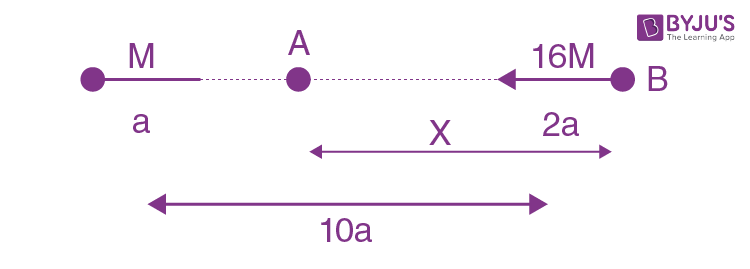A → Where Fnet = 0

[G (16M) (m)] / x2 = [G (M) (m) / (10a - x)2]

x = 8a

So if the particle reaches A it will automatically reach the smaller planet.

Now E – C b/w B and A.

[1 / 2] mv2 – [{G (16M) (m)} / 2a] – [{GMm / 8a}] = [{–G (16M) (m)} / 8a] – [{GMm / 2a}]

V = (3 / 2) √5GM / a

Question 17: In the figure shown, the current in the 10 V battery is close to: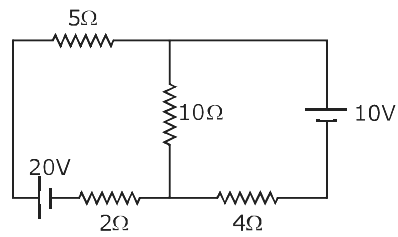1. 1) 0.21 A from positive to the negative terminal
2. 2) 0.36 A from negative to the positive terminal
3. 3) 0.42 A from positive to the negative terminal
4. 4) 0.71 A from positive to the negative terminal

Solution: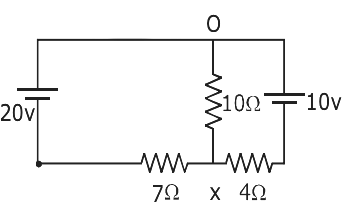[x + 20] / 7 + [x + 10] / 4 + [x / 10] = 0

x = - 1500 / 138 = -10.87

The current through 10 v.

i = [10.87 - 10] / 4 => 0.21 Amp.

Question 18: A student measuring the diameter of a pencil of circular cross-section with the help of a vernier scale records the following four readings 5.50 mm, 5.55 mm, 5.45 mm, 5.65 mm. The average of these four readings is 5.5375 mm and the standard deviation of the data is 0.07395 mm. The average diameter of the pencil should therefore be recorded as:

1. 1) (5.54 ± 0.07) mm
2. 2) (5.5375 ± 0.0740) mm
3. 3) (5.5375 ± 0.0739) mm
4. 4) (5.538 ± 0.074) mm

Solution:

The significant rule says that reading should have the same significant figure as that of the reading given.

5.5375 → rounded to 5.54

Question 19: Given the masses of various atomic particles mp = 1.0072 u, mn = 1.0087 u, me = 0.000548 u, mv[bar] = 0, md = 2.0141 u, where p ≡ proton, n ≡ neutron, e ≡ electron, v [bar] ≡ antineutrino and d ≡ deuteron. Which of the following processes is allowed by momentum and energy conservation?

1. 1) n + n deuterium atom (electron bound to the nucleus)
2. 2) e+ + e → γ
3. 3) p → n + e+ + v [bar]
4. 4) n + p → d + γ

Solution:

(because n + p → d)

(because e + e → γ )

(because mass ↑)

Question 20: A particle moving in the xy plane experiences a velocity-dependent force, where vx and vy are the x and y components of its velocity v. If a is the acceleration of the particle, then which of the following statements is true for the particle?

1. 1) The kinetic energy of the particle is constant in time.
2. 2) quantity v × a is constant in time
3. 3) quantity v . a is constant in time
4. 4) F arises due to a magnetic field

Solution:

Given F = k (Vyi + Vx j)

ma = k (Vyi + Vx j)

ax = kvy / m, ay = kvx / m

Option -1 is incorrect. (K.E. ≠ constant)

Option -2 is correct.

V × a = 0; a = kv / m because v and a are in the same direction.

Option - 3 → v . a = (k / m) [vx2 + vy2] (incorrect)

Option - 4 incorrect.

Question 21: A Young's double-slit experiment is performed using monochromatic light of wavelength λ. The intensity of light at a point on the screen, where the path difference is λ, is K units. The intensity of light at a point where the path difference is λ / 6 is given by nK / 12, where n is an integer. The value of n is __________.

Solution:

From the first case,

Inet = 4I cos2 [Δɸ / 2]

∴ Δɸ = [2π / λ] * λ ⇒ 2π

Inet = 4I = k (given)

From the second case,

Inet = 4I cos2 [Δɸ / 2]

∴ Δɸ = [2π / λ] * [λ / 6] ⇒ π / 3

Inet = 4I × (3 / 4) ⇒ (3 / 4) k = nk / 12

n = 9

Question 22: The centre of mass of the solid hemisphere of radius 8 cm is x from the centre of the flat surface. Then the value of x is __________.

Solution: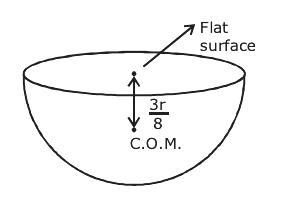As we know c.o.m. or hemisphere = 3r / 8

r = 8cm (given) ⇒ (3 * 8) / 8 ⇒ 3cm

Question 23: The output characteristics of a transistor is shown in the figure. When VCE is 10V and IC = 4.0 mA, then the value of βac is __________.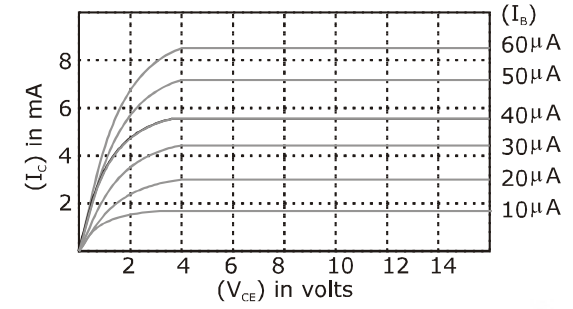Solution:

β = ΔIC / ΔIB

ΔIB = 30 - 20 IC = 4mA (reference value given)

= 10 μA

ΔIC = = 4.5 – 3

= 1.5mA

β = [1.5 × 10-3] / [10 × 10-6]

= 150

Question 24: An engine operates by taking a monatomic ideal gas through the cycle shown in the figure. The percentage efficiency of the engine is close to __________.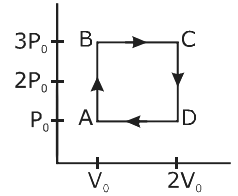Solution:

% efficiency of Carnot engine η = (W / Q) × 100

Work = Area of ABCD = (2P0) / (V0)

Heat = QAB + QBC (input)

QAB = isochoric process

= ncvΔT ∴ PV = nRT

⇒ ncv (TB - TA) T = PV / nR

QAb = 1 × (3 / 2) k (3P0V0 - P0V0) ⇒ 3P0V0

QBC = isobaric process

= nCpΔT

⇒ 1 × (5 / 2) k (6P0V0 - 3P0V0) ⇒ 7.5P0V0

η = {2P0V0 / [3P0V0 + 7.5P0V0]} × 100 ≈ 19%

Question 25: In a series LR circuit, power of 400W is dissipated from a source of 250 V, 50 Hz. The power factor of the circuit is 0.8. In order to bring the power factor to unity, a capacitor of value C is added in series to the L and R. Taking the value of C as (n / 3π) μF, then the value of n is __________.

Solution:

In the first case, the power factor of LR CKT is

cos ɸ = 0.8 = R / √R2 + XL2

where √R2 + XL2 = z ---- (1)

∴ P = VI cosθ

⇒ 400 = (250)2 × (R / Z2)

400 = (250)2 × (0.8 / z)

z = 125 ---- (2)

R = [(250)2 × 0.8 × 0.8] /  ⇒ 100Ω ---- (3)

From (1), (2) and (3),

(100)2 + XL2 = (125)2

XL2 = 15625 10000

XL2 = 5625

XL = 75 ---- (4)

In the second case, it is given that power factor = 1 that means

XL = XC(Resonance condition)

XL = 1 / ωc ⇒ 75 = 1 / {[2πF] C}

C = 1 / {[2πF] 75}

C = {1 / [2π × 50 × 75]} F ----- (5)

C = (n / 3π) μF given ---- (6)

From (5) & (6),

{1 / [2π × 50 × 75]} = [n × 10-6] / 3π

n = 106 / 7500 ⇒ [3 × 104] / 75 ⇒ 30000 / 75

n = 400

### JEE Main 2020 Physics Paper With Solutions Shift 2 September 6